# 怎样加减正数与负数

## 数可以是正或负：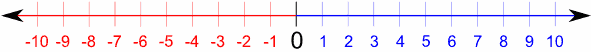负数 (−) 正数 (+) （这是 实数直线，去这里了解如何使用实数直线)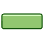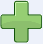"−" 是 负号 "+" 是 正号

Example: 5 就是 +5

"正2 加 正3 等于 正5"

"正6 减 正3 等于 正3"

## 气球 和 重量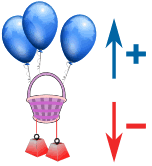这个篮子与气球和重物系在一起： 气球向上拉 （正） 重物向下拉 （负）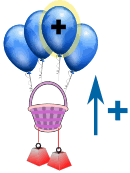你可以加气球 （你在 加正 值） 篮子被拉上去了 （正）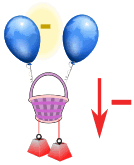你可以拿走气球 （你在 减 正 值） 篮子被拉下来了 （负）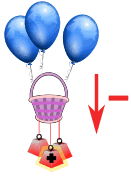你可以加重物 （你在 加 负 值） 篮子被拉下来了 （负）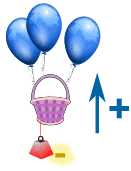你也可以拿走重物 （你在 减 负 值） 篮子被拉上去了 （正）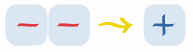减负数和加是一样的

### 例子： 6 − (−3) 是什么？

6−(−3) = 6 + 3 = 9

### 例子： 14 − (−4) 是什么？

14−(−4) = 14 + 4 = 18

## 正数与负数一起。。。。。。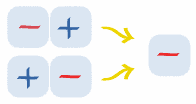减正数 或 加负数 是 减

6−(+3) = 6 3 = 3

### 例子： 5 + (−7) 是什么？

5+(−7) = 5 7 = −2

## 规则：

规则 例子 +(+)两个相同的符号成为一个 正号 3+(+2) = 3 + 2 = 5 6−(−3) = 6 + 3 = 9两个不同的符号成为一个 负号 7+(−2) = 7 − 2 = 5 8−(+2) = 8 − 2 = 6

### 例子： 5+(−2) 是什么？

+(−) 是 不同 的符号 （它们不是同一个符号），所以它们成为一个 负号

5+(−2) = 5 2 = 3

### 例子： 25−(−4) 是什么？

−(−) 是相同 的符号，所以它们成为一个 正号

25−(−4) = 25+4 = 29

### 例子： −6+(+3) 是什么？

+(+) 是 相同 的符号，所以它们成为一个 正号

−6+(+3) = −6 + 3 = −3

## 来玩玩！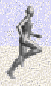玩玩 凯西跑手，你需要熟悉正负的规则才能赢！

## 一个符合常理的解释## 再一个符合常理的解释

 + + ⇒ + 朋友的朋友是朋友 + − ⇒ − 朋友的敌人是敌人 − + ⇒ − 敌人的朋友是敌人 − − ⇒ + 敌人的敌人是朋友

## 银行的例子

### 去年银行误扣了 \$10 ，他们要做个调整。

\$80−(−\$10) = \$80 + \$10 = \$90